# Chebyshev inequality in probability theory

(diff) ← Older revision | Latest revision (diff) | Newer revision → (diff)

Bienaymé–Chebyshev inequality

2010 Mathematics Subject Classification: Primary: 60E15 [MSN][ZBL]

An inequality in probability theory that gives a bound on the probability of deviation of a given random variable from its mathematical expectation in terms of its variance. Letbe a random variable with finite mathematical expectation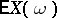and variance. Chebyshev's inequality states that for anythe probability of the eventdoes not exceed, or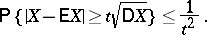(1)

This inequality was discovered independently by I. Bienaymé (1853) and P.L. Chebyshev (1866). In modern literature this inequality is usually referred to as Chebyshev's inequality, possibly because the name of Chebyshev is associated with an application of it in the proof of the law of large numbers (a theorem of Chebyshev).

Chebyshev's inequality is a representative of a whole class of inequalities of this type, the simplest of which asserts that for a non-negative random variablewith finite mathematical expectation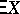,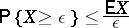(2)

(this is sometimes called Markov's inequality). This implies an inequality for arbitrary random variables, which depends on the moments:(for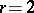this is just the Chebyshev inequality), and also the more general inequality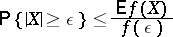(3)

for a non-negative even function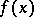that is non-decreasing for positive. Inequality (3) indicates a way to obtain new inequalities of the same type, for example the exponential inequality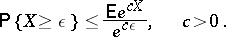It has become traditional to consider all these inequalities to be of Chebyshev type, and even to call them Chebyshev inequalities. There is a general principle for obtaining Chebyshev inequalities by imposing conditions on the moments, based on the use of the system of Chebyshev polynomials (cf. [KS]). For arbitrary random variables the Chebyshev inequalities give precise and best possible bounds, but in certain concrete situations these bounds can be improved. For example, ifhas a unimodal distribution with modecoinciding with the mathematical expectation, then Gauss' inequality holds: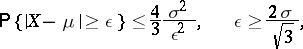where.

The importance of Chebyshev's inequality in probability theory lies not so much in its exactness, but in its simplicity and universality. Chebyshev's inequality and its modifications, applied to sums of random variables, played a large part in the proofs of various forms of the law of large numbers and the law of the iterated logarithm. Chebyshev's inequality for sums of independent random variables has been subject to generalization and improvement in two different directions. The first of these is connected with the transition from the Chebyshev inequality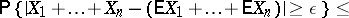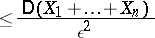to the significantly stronger inequalitywhich was proved by A.N. Kolmogorov and applied by him to prove the strong law of large numbers (cf. Kolmogorov inequality).

The second direction is concerned with replacing the power in Chebyshev's inequality by something with exponential decay, and leads to the Bernshtein–Kolmogorov inequality: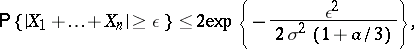where,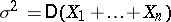,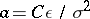(cf. Bernstein inequality). Such improvements of Chebyshev inequalities are obtained under additional restrictions on the summands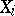.

Multi-dimensional analogues have been obtained of some of the inequalities stated here (cf. [P]).